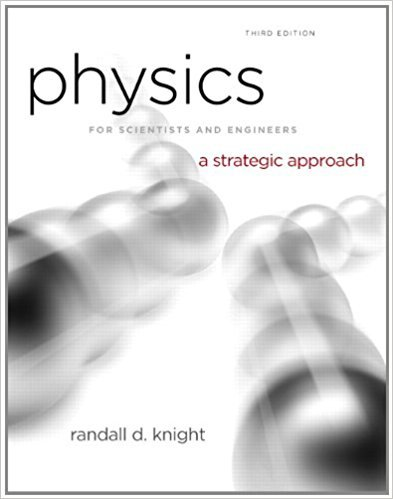×
Get Full Access to Physics For Scientists And Engineers: A Strategic Approach With Modern Physics - 3 Edition - Chapter 5 - Problem 44p
Get Full Access to Physics For Scientists And Engineers: A Strategic Approach With Modern Physics - 3 Edition - Chapter 5 - Problem 44p

×

# Solved: describe a situation. For each problem, draw aISBN: 9780321740908 69

## Solution for problem 44P Chapter 5

Physics for Scientists and Engineers: A Strategic Approach with Modern Physics | 3rd Edition

• Textbook Solutions
• 2901 Step-by-step solutions solved by professors and subject experts
• Get 24/7 help from StudySoup virtual teaching assistantsPhysics for Scientists and Engineers: A Strategic Approach with Modern Physics | 3rd Edition

4 5 1 269 Reviews
22
3
Problem 44P

Problem 44P

Problem describe a situation. For each problem, draw a motion diagram, a force identification diagram, and a freebody diagram.

A rocket is being launched straight up. Air resistance is not negligible.

Step-by-Step Solution:

Solution 44P

Step 1 of 3

Motion diagram

As the rocket is moving up, its velocity keeps on increasing with time The motion diagram is shown as below.Step 2 of 3

Step 3 of 3

##### ISBN: 9780321740908

Since the solution to 44P from 5 chapter was answered, more than 333 students have viewed the full step-by-step answer. The answer to “describe a situation. For each problem, draw a motion diagram, a force identification diagram, and a freebody diagram.A rocket is being launched straight up. Air resistance is not negligible.” is broken down into a number of easy to follow steps, and 29 words. This full solution covers the following key subjects: diagram, identification, describe, air, draw. This expansive textbook survival guide covers 17 chapters, and 1439 solutions. This textbook survival guide was created for the textbook: Physics for Scientists and Engineers: A Strategic Approach with Modern Physics, edition: 3. The full step-by-step solution to problem: 44P from chapter: 5 was answered by , our top Physics solution expert on 08/30/17, 04:34AM. Physics for Scientists and Engineers: A Strategic Approach with Modern Physics was written by and is associated to the ISBN: 9780321740908.

Unlock Textbook Solution